Precalculus : Find the Equations of Vertical Asymptotes of Tangent, Cosecant, Secant, and Cotangent Functions

Example Questions

Example Question #1 : Find The Equations Of Vertical Asymptotes Of Tangent, Cosecant, Secant, And Cotangent Functions

Given the function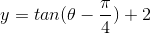, determine the equation of all the vertical asymptotes across the domain.  Letbe an integer.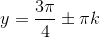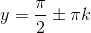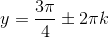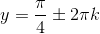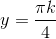Explanation:

For the function, it is not necessary to graph the function.  The y-intercept does not affect the location of the asymptotes.

Recall that the parent function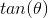has an asymptote at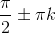for everyperiod.

Set the inner quantity of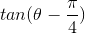equal to zero to determine the shift of the asymptote.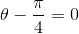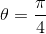This indicates that there is a zero at, and the tangent graph has shifted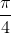units to the right.  As a result, the asymptotes must all shiftunits to the right as well.  The period of the tangent graph is.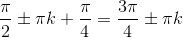All Precalculus Resources